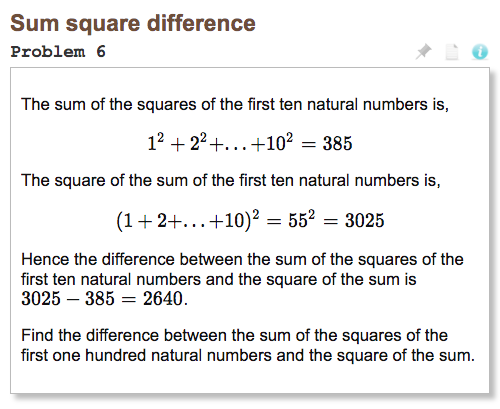# Project Euler Problem 6: Sum Square DifferenceProblem 6 has a brute force solution and an elegant formula solution that calculates the answer directly. But the brute force solution is good enough and the formula is obscure enough that I wouldn’t have found it without googling so we’ll focus on the brute force solution.

As always, spend some time with the problem if you haven’t yet.

## Brute Forcing it

All the numbers involved in this problem are small enough to quickly calculate:

limit = 100
integers = range(1, limit + 1)
sum_of_squares = sum([x ** 2 for x in integers])
square_of_sum = sum(integers) ** 2
print(square_of_sum - sum_of_squares)


You could get clever and jam all that into one line, but it’d be less readable & maintainable:

print(sum(range(1, 101)) ** 2 - sum([x ** 2 for x in range(1, 101)]))


I feel the temptation to get clever like that a lot. I’ve learned future me is usually better off if I avoid that temptation.

## The Efficient Solution

It’s not my jam and I didn’t figure it out on my own (I just used the brute force solution) so I’ll avoid deriving the formula (you can read about it in depth here if you like), but for the series of squares:

$$1^2{,\ } 2^2{,\ } 3^2{,\ } 4^2{,\ } 5^2…$$

there’s a formula to calculate the sum of the first n terms:

$$\sum_{i=1}^{n} i^2 = \frac{n^3}{3} + \frac{n^2}{2} + \frac{n}{6}$$

And of course we know the sum of 1 to 100 is a good old arithmetic progression:

$$\sum_{a_{1}}^{a_{n}} = \frac{n(a_{1} + a_{n})}{2}$$

We can combine those as:

$$Answer = \left( \frac{100^3}{3} + \frac{100^2}{2} + \frac{100}{6} \right) - \left( \frac{100(1 + 100)}{2} \right)^2$$

In code:

limit = 100
sum_of_squares = (limit * (1 + limit) / 2) ** 2
square_of_sum = (limit ** 3) / 3 + (limit ** 2) / 2 + limit / 6
print(sum_of_squares - square_of_sum)


Look ma, no iteration.

See an issue on this page? Report a typo, bug, or give general feedback on GitHub.